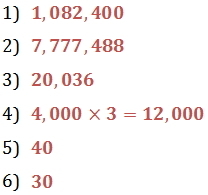Lesson - Multiplication

Multiplication

Estimating Multiplication

At times, you will want to estimate the product of two numbers. Sometimes an exact answer is not needed.  Estimating may also help you check if a calculator-generated answer is correct.

To estimate a product using front-end estimation, round each number so that all the digits are zero except the first digit.  Then multiply.

Example

Estimate the product of 3267 x 389.

3,000 x 400 = 1,200,000

3267 x 389 = 1270863

Finding the LCM (Least Common Multiple)

Multiples of a number are the products of that number and other numbers such as 1, 2, 3, 4, 5, ....

5 x 1 = 5
5 x 2 = 10
5 x 3 = 15
5 x 4 = 20
The multiples of 5 are 5, 10, 15, 20, 25, 30....
5 x 5 = 25
5 x 6 = 30

..... and so on

A number that is a multiple of two or more numbers is a common multiple of those numbers.

The least common multiple (LCM) is the smallest common multiple of two or more numbers. One way to find the LCM of two numbers is by listing multiples and identifying the smallest one in common.

Example

Find the LCM of 4 and 6.

STEP 1

List some multiples of 4 and 6. Make note of the common multiples, the numbers that appear in both lists.

4:  4, 8, 12, 16, 20, 24, 28, …
6:  6, 12, 18, 24, 30, 36, 42, …

STEP 2

Identify the least common multiple.
The least common multiple is 12.
The LCM of 4 and 6 is 12.

Practice

1.  Calculate the product, and then check your answer by using a calculator.

5,280 x 205

2.  Calculate the product, and then check your answer by using a calculator.

25,008 x 311

3.  How much is 5,009 multiplied by 4?

4.  Lincoln's Sub Shop sold 4,000 sandwiches during the month July.  During August they sold almost three times as many sandwiches.  About how many sandwiches were sold in August?

5.  Find the LCM of 5 and 8.

6.  Find the LCM of 5, 10 and 15.